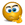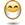# Change the range of pivot table dynamically

## Excel Help for Change The Range Of Pivot Table Dynamically in Charting and Performing Technical Analysis

### Change The Range Of Pivot Table Dynamically

Rate this:
(3/5 from 1 vote)Dear FriendsGreetings To You,I want to update the range value of pivot table dynamically e.g.Initially I have created pivot table on 10 rows of Sheet1.At run time the rows in the Sheet1 have changed lets say now we have 20 rows.The pivot table should refer to 20 rows (Sheet1 is created dynamically).Advance thanks kiran Posted by guntikirankumar on 09 Dec 2010
 Replies - Displaying 1 to 3 of 3 Order Replies By: Most Recent | Chronological | Highest RatedRate this:
You can change the pivot table source data dynamically within a VBA macro.
In Sheet1 you first need to get the last row number such as:

VBA Code:
 Dim lastrow as IntegerSheets("Sheet1").SelectRange("A1").SelectSelection.End(xlDown).Selectlastrow = ActiveCell.Row

Then use the last row number to refresh the pivot table source data range such as:

VBA Code:
 ActiveSheet.PivotTables("PivotTable1").ChangePivotCache ActiveWorkbook.PivotCaches.Create(SourceType:=xlDatabase, SourceData:="Sheet1!R1C1:R" & lastrow & "C3")

Posted by onRate this:
(3/5 from 1 vote)
Hi,

I believe the below code should allow users to update multiple pivots in multiple worksheets by using a dynamic range variable determined from using a Last function.  The code below should also allow you to specify which datafields the user would like included.

My code is below:

VBA Code:
 Sub Refresh_All_Pivots() On Error GoTo err_handler Application.ScreenUpdating = False Dim field1 As StringDim field2 As StringDim field3 As StringDim field4 As StringDim field5 As StringDim field6 As StringDim field7 As StringDim field8 As String Dim ws As WorksheetDim Pt As PivotTableDim pf As PivotField Dim rng As RangeDim lastcol As LongDim LastRow As Long For Each ws In ActiveWorkbook.Worksheetsws.Activate    For Each Pt In ws.PivotTables               Set rng = Range("A4").currentregion        LastRow = Last(1, rng)    lastcol = Last(2, rng)     field1 = rng.Cells(1, (lastcol) - 7).Text     field2 = rng.Cells(1, (lastcol) - 6).Text        field3 = rng.Cells(1, (lastcol) - 5).Text    field4 = rng.Cells(1, (lastcol) - 4).Text    field5 = rng.Cells(1, (lastcol) - 3).Text    field6 = rng.Cells(1, (lastcol) - 2).Text    field7 = rng.Cells(1, (lastcol) - 1).Text    field8 = rng.Cells(1, (lastcol)).Text               Pt.SourceData = Range("A4").currentregion.Address(True, True, xlR1C1, True)                                    For Each pf In Pt.DataFields            pf.Orientation = xlHidden        Next pf                                                  With Pt.PivotFields(field1)                .Orientation = xlDataField                .Caption = "Sum of" & " " & field1                .Function = xlSum            End With                                            With Pt.PivotFields(field2)                .Orientation = xlDataField                .Caption = "Sum of" & " " & field2                .Function = xlSum            End With                            With Pt.PivotFields(field3)                .Orientation = xlDataField                .Caption = "Sum of" & " " & field3                .Function = xlSum            End With                            With Pt.PivotFields(field4)                .Orientation = xlDataField                .Caption = "Sum of" & " " & field4                .Function = xlSum            End With                            With Pt.PivotFields(field5)                .Orientation = xlDataField                .Caption = "Sum of" & " " & field5                .Function = xlSum            End With                            With Pt.PivotFields(field6)                .Orientation = xlDataField                .Caption = "Sum of" & " " & field6                .Function = xlSum            End With                            With Pt.PivotFields(field7)                .Orientation = xlDataField                .Caption = "Sum of" & " " & field7                .Function = xlSum            End With             With Pt.PivotFields(field8)            .Orientation = xlDataField            .Caption = "Sum of" & " " & field8            .Function = xlSum            End With        Pt.RefreshTableErrorhandling:          Next PtNext ws ActiveWorkbook.ShowPivotTableFieldList = FalseApplication.ScreenUpdating = True Sheet1.Activate Exit Sub err_handler:MsgBox "One or more of the Pivot tables have experienced an error." & vbCrLf & vbCrLf & _    "Please look through the workbook to determine where the error has occurred.", _    vbOKOnly, "Error with Refreshing Pivots" Resume Errorhandling End Sub

Just completed this (I think) so thought I would share.

All ears if anyone encounters any problems?

Thanks

Carl

Posted by onRate this: (3/5 from 1 vote) rather than use vba you can use the offset function and make a named range.  There's a site called [http://www.tips-for-excel.com/] that covers pivot tables like this if I remember correctly Posted by wilsonkiw on 22 Jun 2011
 Displaying page 1 of 1

 Find relevant Excel templates and add-ins for Change the range of pivot table dynamically in the Excel Business Solutions Directory